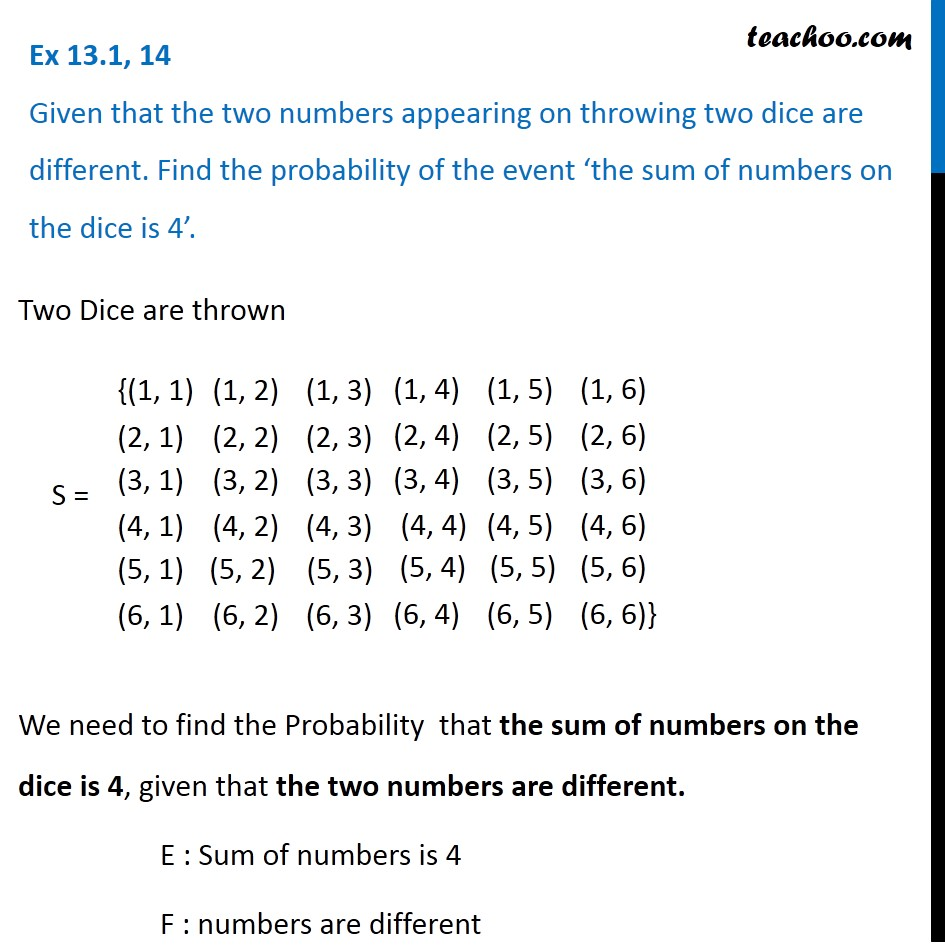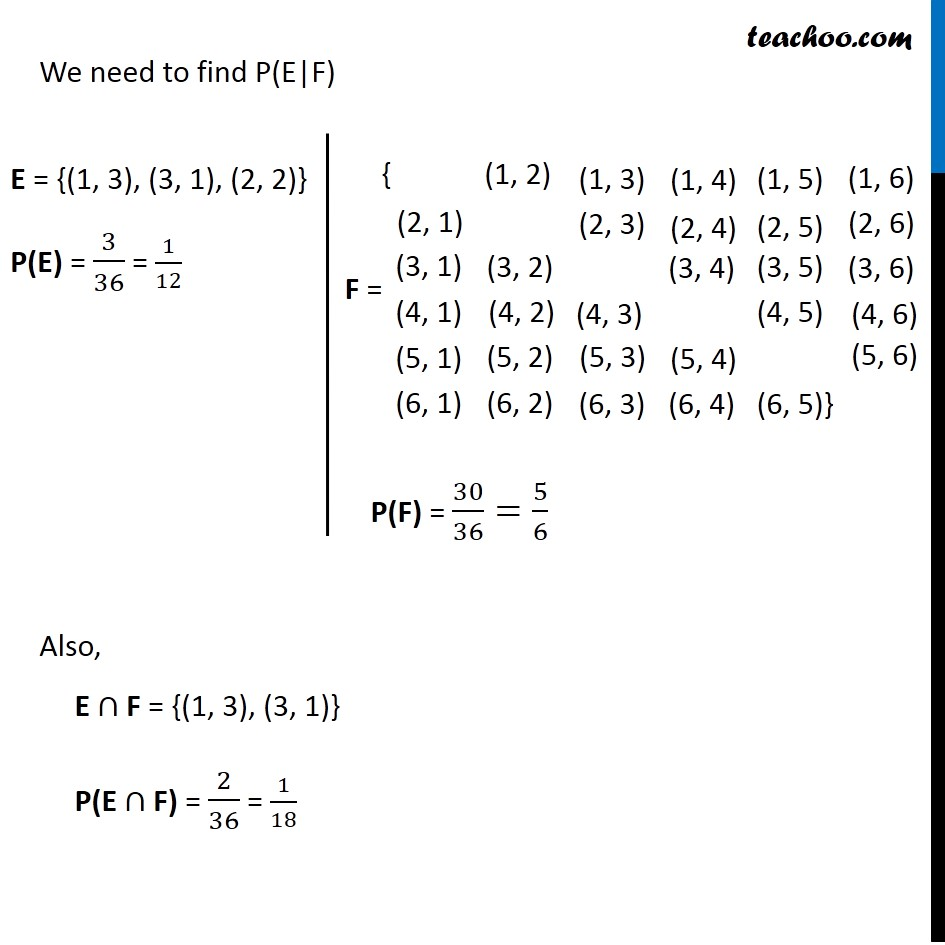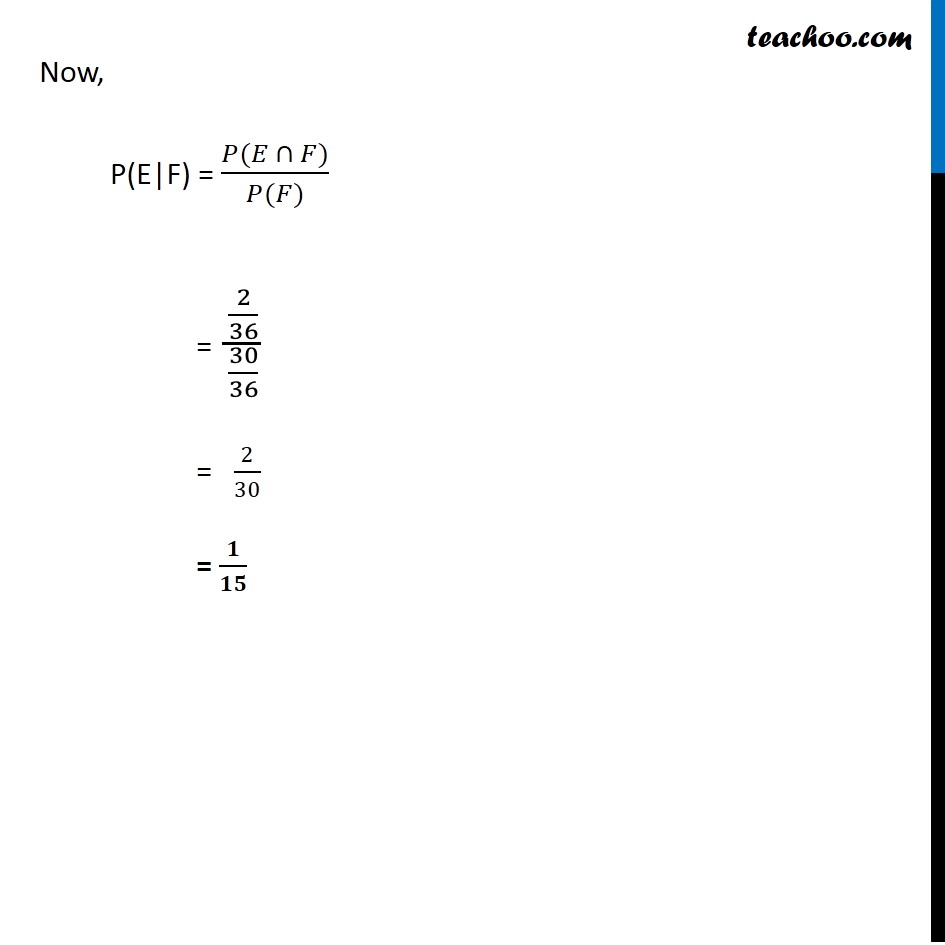Ex 13.1

Chapter 13 Class 12 Probability
Serial order wiseLearn in your speed, with individual attention - Teachoo Maths 1-on-1 Class

### Transcript

Ex 13.1, 14 Given that the two numbers appearing on throwing two dice are different. Find the probability of the event ‘the sum of numbers on the dice is 4’.Two Dice are thrown We need to find the Probability that the sum of numbers on the dice is 4, given that the two numbers are different. E : Sum of numbers is 4 F : Numbers are different We need to find P(E|F) Also, E ∩ F = {(1, 3), (3, 1)} P(E ∩ F) = 2/36 = 1/18 Event E E = {(1, 3), (3, 1), (2, 2)} P(E) = 3/36 = 1/12 Event F P(F) = 30/36=5/6 Now, P(E|F) = (𝑃(𝐸 ∩ 𝐹))/(𝑃(𝐹)) = (2/36)/(30/36) = 2/30 = 𝟏/𝟏𝟓